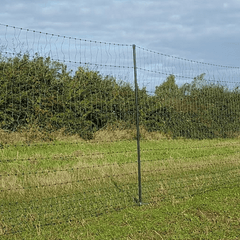Electric netting is renowned for being resource hungry meaning that a given net requires a certain strength energiser to be able to run it. All reputable energisers (of course ours are totally reputable) will give figures for how many nets will be run by that energiser. This is generally referring to standard 1 meter high by 50 meter long nets.

If we look at the popular Farmer N00, it will power about 12000 meters of single wire fencing but will only run 7 nets. If we multiply the nets out we will find that there is only 5450 meters of wire in the 7 nets suggested so there is a massive discrepancy between the two.

Where a company only quotes a length of fencing it is possible to be misled to assume that a large number of nets may be charged by a particular energiser. In the case of the example of the Farmer N100, this would be 27 nets which is not possible. If no figures are quoted then it will be prudent to assume only 40% of that figure quoted for single wire fencing is available for netting.

Why does this Happen?

An electric fence relies upon the energiser sending down the wire a high voltage charge for only 1/300 of a second, once a second *, When that happens a magnetic field is created around that wire. In netting these wires are all close together so the magnetic fields around each wire interact with each other so increasing the resistance. This continually happens as the magnetic field collapses and is re-created every time a charge is sent down the line. This is highlighted by what is known as the Hall effect.

The general explanation of the theory of the Hall effect and the change of resistance in a magnetic field expresses these quantities in terms of several integrals over the surface of the Fermi distribution. Measured with a constant current, resistance increases under the influence of a magnetic field, and that the increase depends on the strength of the field and its direction with reference to the current in the wire. If the current traversing the bismuth is oscillatory, the resistance has a value O outside the magnetic field, or in a field in which the lines of force are parallel to the wire which is less than R. If, however, the wire is perpendicular to the lines of force of a field greater than 6000 C.G.S. units, the resistance O is greater than R; the difference O – R increases from this point rapidly as the strength of the field increases.  The difference between the resistances to an increasing and decreasing current increases with the rate of change in the strength of the current and this difference is more marked with strong currents than with weak. (Hope you understood that)Not only is resistance affected by the oscillation of the electric current, the type of conductor also affects the reaction. A Copper/Zinc alloy will carry more current but be less affected by the magnetic affect than those made of Stainless Steel. High diamagnetism and strong resistance go together. Stainless Steel has a higher resistance coefficient than a Copper/Zinc alloy in filaments of the same diameter. For this reason nets should be quoted with the conductivity of the twine.

### Agrisellex nets are all less than 1 Ohm/meter with the Ultimate Nets being 0.75 Ohms/meter.

Ambient temperature has a further effect. As the temperature increases so does the resistance in the wire increase which then has a greater effect on the magnetism resulting in a greater in-line resistance. This "greater" is actually very small in real terms over temperatures normally encountered (about 0.02 Ohms increase between 5 and 25 degrees C.) so is discounted and ignored.

The best part of this is the cost of Electric Fence energisers is not relative to their strength. Double the strength does not double the price, normal increase is about 20% for a 100% increase in energy supply.

#### Get a Wimp energiser and you WILL have a wimp fence.

* These figures do vary between both models and manufacturers.